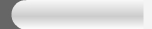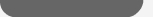Copyright (c) Newday Com. Ltd. All rights reserved-2001-2008### By the end of this lesson, learners should be able to:

• Understand definition of a function
• Use Excel 2003 functions in calculations
• Access Excel 2003 functions
• Find the sum of a range of data

### Using Functions

A function is a pre-defined formula that helps perform common mathematical functions. Functions save you the time of writing lengthy formulas. You could use an Excel function called Average, for example, to quickly find the average of range of numbers. Or you could use the Sum function to find the sum of a cell range. Excel 2003 contains many different functions.

Each function has a specific order, called syntax, which must be strictly followed for the function to work correctly.

Syntax Order:

1. All functions begin with the = sign.
2. After the = sign define the function name (e.g., Sum).
3. One or more arguments-numbers, text or cell references-enclosed by parentheses. If there is more than one argument, separate each by a comma.

An example of a function with one argument that adds a range of cells, B3 through B10:An example of a function with more than one argument that calculates the average of numbers in a range of cells, B3 through B10, and C3 through C10:Excel literally has hundreds of different functions to assist with your calculations. Building formulas can be difficult and time-consuming. Excel's functions can save you a lot of time and headaches.

### Excel's Different Functions

There are many different functions in Excel 2003. Some of the more common functions include:

##### Statistical Functions:
• SUM - summation adds a range of cells together.
• AVERAGE - average calculates the average of a range of cells.
• COUNT - counts the number of chosen data in a range of cells.
• MAX - identifies the largest number in a range of cells.
• MIN - identifies the smallest number in a range of cells.
##### Financial Functions:
• Interest Rates
• Loan Payments
• Depreciation Amounts
##### Date and Time functions:
• DATE - Converts a serial number to a day of the month
• Day of Week
• DAYS360 - Calculates the number of days between two dates based on a 360-day year
• TIME - Returns the serial number of a particular time
• HOUR - Converts a serial number to an hour
• MINUTE - Converts a serial number to a minute
• TODAY - Returns the serial number of today's date
• MONTH - Converts a serial number to a month
• YEAR - Converts a serial number to a yearYou don't have to memorize the functions but should have an idea of what each can do for you.

### Finding the Sum of a Range of Data

The AutoSum function allows you to create a formula that includes a cell range-many cells in a column, for example, or many cells in a row.##### To Calculate the AutoSum of a Range of Data:
• Type the numbers to be included in the formula in separate cells of column B (Ex: type 128 in cell B2, 345 in cell B3, 243 in cell B4, 97 in cell B5 and 187 cell B6).
• Click on the first cell (B2) to be included in the formula.
• Using the point-click-drag method, drag the mouse to define a cell range from cell B2 through cell B6.
• On the Standard toolbar, click the Sum button.
• The sum of the numbers is added to cell B7, or the cell immediately beneath the defined range of numbers.
• Notice the formula, =SUM(B2:B6), has been defined to cell B7.### Finding the Average of a Range of Numbers

The Average function calculates the average of a range of numbers. The Average function can be selected from the AutoSum drop-down menu.

##### To Calculate the Average of a Range of Data:
• Type the numbers to be included in the formula in separate cells of column B (Ex: type 128 in cell B2, 345 in cell B3, 243 in cell B4, 97 in cell B5 and 187 cell B6).
• Click on the first cell (B2) to be included in the formula.
• Using the point-click-drag method, drag the mouse to define a cell range from cell B2 through cell B6.
• On the Standard toolbar, click on the drop-down part of the AutoSum button.• Select the Average function from the drop-down Functions list.• The average of the numbers is added to cell B7, or the cell immediately beneath the defined range of numbers.
• Notice the formula, =AVERAGE(B2:B6), has been defined to cell B7.### Accessing Excel 2003 Functions

##### To Access Other Functions in Excel:
• Using the point-click-drag method, select a cell range to be included in the formula.
• On the Standard toolbar, click on the drop-down part of the AutoSum button.
• If you don't see the function you want to use (Sum, Average, Count, Max, Min), display additional functions by selecting More Functions.• The Paste Function dialog box opens.
• There are three ways to locate a function in the Insert Function dialog box:

You can type a question in the Search for a function box and click GO, or

You can scroll through the alphabetical list of functions in the Select a function field, or

You can select a function category in the Select a category drop-down list and review the corresponding function names in the Select a function field.• Select the function you want to use and then click the OK button.

### Challenge!

• Open your Monthly Budget file.
• Type the following in Row 1:
• February in D1.
• March in E1.
• April in F1.
• May in G1.
• June in H1.
• July in I1.
• August in J1.
• September in K1.
• October in L1.
• November in M1.
• December in N1.
• Total in O1.
• Type the amount of your expenses in each cell in Column D (cells 2 through 17), just like you did with Column C in a previous challenge.
• Delete the number in C15.
• Type a function in cell C15 that adds the range of cells, C2 through C14.• Fill the formula from C15 to D15 through O15.
• Type your Income for the month of February in D16.
• Type a formula in O17 that adds your savings for the year. Since you have only entered data for the month of January and February, this amount indicates your savings for the two months.
• Save and close the Monthly Budget spreadsheet.

 NOTICE BOARD 1  Student should make sure to login to class discussion board to view the neccessary information about his or her progress.  Don,t forget to log out when you are about to leave the classNOTICE BOARD 2 Students Should not forget to send his or her registration password and User name to newday.com@gmail.com For future refrence.NOTICE BOARD 3 Student should make sure to subscribe to newsletter of this site for useful information.MORE ADVANCE COURSE  Microsoft Access  Peachtree Account  Adobe Photoshop  ProgrammingENGINNERING COURSE  Café Management  Repair and Maintenance  Electronic Basic  Networking